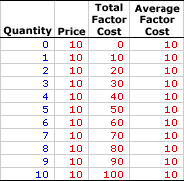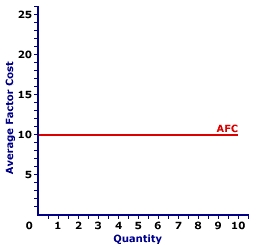Wednesday  June 26, 2019
 AmosWEB means Economics with a Touch of Whimsy!LAW: Generally accepted, verified, fundamental principle of nature. Laws have been tested and verified through the scientific method. As a house is constructed from concrete, lumber, and nails, a theory is constructed from laws. To be a fundamental law of nature, a principle must capture a cause-and-effect relationship about the workings of the world.AVERAGE FACTOR COST, PERFECT COMPETITION:

Total factor cost per unit of factor input employed by a perfectly competitive firm in the production of output, found by dividing total factor cost by the quantity of factor input. Average factor cost, abbreviated AFC, is generally equal to the factor price. However, using the longer term average factor cost makes it easier to see the connection to related terms, including total factor cost and marginal factor cost.
Average factor cost is the per unit opportunity cost incurred by a perfectly competitive firm from the employment of a given resource. It plays a key role in the study of factor markets. Average factor cost is constant (that is, it does not change if the quantity of the factor input changes) for a firm buying factors through a perfectly competitive factor market. For a monopsony buyer, average factor cost increases as the quantity of factor input increases. The constant or increasing nature of average factor cost is a prime indication of the market control of a firm.

Whichever market structure is involved, average factor cost is calculated as the total factor cost divided by the quantity of the factor purchased, as illustrated by this equation:

 average factor cost = total factor costfactor quantity
At times, it is useful to turn this equation around and calculate total factor cost from average factor cost:
 total factor cost = average factor cost x factor quantity
If the firm is hiring the factor in a perfectly competitive factor market, then the factor price is fixed or constant and so too is average factor cost. If the firm is hiring the factor in an imperfectly competitive factor market, best illustrated by monopsony, then the factor price increases with larger factor quantities and so too does average factor cost.

Perfect competition is a market structure with a large number of small participants (buyers and sellers). The good exchanged in the market is identical, regardless of who sells or who buys. Participants have perfect knowledge and perfect mobility into and out of the market. These conditions mean perfectly competitive buyers are price takers, they have no market control and must pay the going market price for all inputs bought.

Average Factor Cost,
Perfect CompetitionThe table to the right summarizes the average factor cost incurred by a hypothetical buyer, Maggie's Macrame Shoppe, for hiring store clerks in a perfectly competitive labor market. Maggie's Macrame Shoppe is one of thousands of small retail stores in the greater Shady Valley metropolitan area that hires labor with identical skills. As such, Maggie pays the going wage for labor.

The first column in the table is the quantity of workers hired, ranging from 0 to 10 workers. The second column is the price Maggie pays for hiring her workers, which is constant at \$10 per worker. The third column is the total factor cost Maggie incurs for hiring various numbers of workers. If Maggie hires only one worker, then she pays only \$10. If she hires five workers, she pays \$50. In each case, total factor cost in column three is calculated as the quantity in the first column multiplied by the price in the second column.

The fourth column then presents the average factor cost incurred by Maggie at each level of employment. These numbers are found by dividing total factor cost in the third column by factor quantity in the first column. For example, the total factor cost of hiring five workers is \$50, resulting in an average factor cost of \$10 per worker.

The two key observations about average factor cost are:

• First, average factor cost is constant. Each level of employment results in the same \$10 average factor cost.

• Second, average factor cost is equal to price at every level of employment. Average factor cost and price are basically two terms for the same concept.
Average Factor Cost Curve,
Perfect CompetitionAverage factor cost is commonly represented by an average factor cost curve, such as the one displayed in the exhibit to the right. This particular average factor cost curve is that for labor hired by Maggie's Macrame Shoppe.

The vertical axis measures average factor cost and the horizontal axis measures the quantity of input (workers). Although quantity on this particular graph stops at 10 workers, the nature of perfect competition indicates it could easily go higher.

First and foremost, the average factor cost curve is horizontal at the going factor price of \$10. This indicates that if Maggie hires 1 worker, then she pays an average factor cost of \$10. Alternatively, if she hires 10 workers, then she pays an average factor cost of \$10. Should she hire 100 workers, then she might move well beyond the graph, but she pays an average factor cost of \$10.

The "curve" is actually a "straight line" because Maggie is a price taker in the labor market. She pays \$10 for each worker whether she hires 1 worker or 10 workers or 100 workers. The constant price is what makes Maggie's average factor cost curve a straight line, and which indicates that Maggie has no market control.

 <= AVERAGE FACTOR COST, MONOPSONY AVERAGE FIXED COST =>Recommended Citation:

AVERAGE FACTOR COST, PERFECT COMPETITION, AmosWEB Encyclonomic WEB*pedia, http://www.AmosWEB.com, AmosWEB LLC, 2000-2019. [Accessed: June 26, 2019].

Check Out These Related Terms...

Or For A Little Background...

And For Further Study...
Search Again?YELLOW CHIPPEROON[What's This?] Today, you are likely to spend a great deal of time watching the shopping channel seeking to buy either a flower arrangement with daisies and carnations for your uncle or a coffee cup commemorating next Thursday. Be on the lookout for the last item on a shelf.Your Complete ScopeNatural gas has no odor. The smell is added artificially so that leaks can be detected."You can't use up creativity. The more you use, the more you have. "-- Maya Angelou, poetICCHInternational Commodities Clearing HouseA PEDestrian's Guide Xtra CreditTell us what you think about AmosWEB. Like what you see? Have suggestions for improvements? Let us know. Click the User Feedback link.| | | | | | | | | | |
| | | |

Thanks for visiting AmosWEB